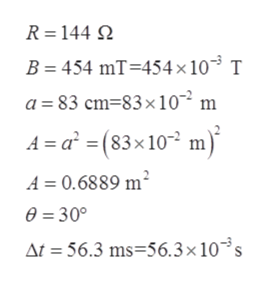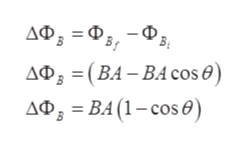# A 144-Ω light bulb is connected to a conducting wire that is wrapped into the shape of a square with side length of 83.0 cm. This square loop is rotated within a uniform magnetic field of 454 mT.What is the change in magnetic flux through the loop when it rotates from a position where its area vector makes an angle of 30° with the field to a position where the area vector is parallel to the field?The loop rotates from a position where its area vector makes an angle of 30° with the field to a position where the area vector is parallel to the field in 56.3 ms. What is the induced current through the light bulb?This square loop is rotated with a frequency of 60 Hz within a uniform magnetic field of 454 mT. This means the loop makes half a revolution in 8.33 ms. What is the induced current in the light bulb when the loop rotates from a position where its area vector is opposite the magnetic field to a position where its area vector is parallel to the magnetic field?

Question
50 views

A 144-Ω light bulb is connected to a conducting wire that is wrapped into the shape of a square with side length of 83.0 cm. This square loop is rotated within a uniform magnetic field of 454 mT.

What is the change in magnetic flux through the loop when it rotates from a position where its area vector makes an angle of 30° with the field to a position where the area vector is parallel to the field?

The loop rotates from a position where its area vector makes an angle of 30° with the field to a position where the area vector is parallel to the field in 56.3 ms. What is the induced current through the light bulb?

This square loop is rotated with a frequency of 60 Hz within a uniform magnetic field of 454 mT. This means the loop makes half a revolution in 8.33 ms. What is the induced current in the light bulb when the loop rotates from a position where its area vector is opposite the magnetic field to a position where its area vector is parallel to the magnetic field?

check_circle

Step 1

Given,help_outlineImage TranscriptioncloseR 144 B 454 mT 454 x 10 T a 83 cm-83x102 m A a(83x 10 m A 0.6889 m2 e=30° At = 56.3 ms-56.3 x 10s fullscreen
Step 2

The change in magnetic flux (ΔΦB) through the loop when it rotates from a position where its area vector makes an angle of 30° with the field to a position where the area vector is parallel to the field can be evaluated as:help_outlineImage TranscriptioncloseДФ, — Ф, - Ф, ДФ, 3 (ВА - ВА cos ®) ДФ, - ВА(1-сos®) fullscreen
Step 3

Plugging the given va...

### Want to see the full answer?

See Solution

#### Want to see this answer and more?

Solutions are written by subject experts who are available 24/7. Questions are typically answered within 1 hour.*

See Solution
*Response times may vary by subject and question.
Tagged in

### Other